The availability of step-by-step ICSE Class 9 Maths Solutions S Chand Chapter 9 Mid-Point and Intercept Theorems Ex 9(A) can make challenging problems more manageable.

## S Chand Class 9 ICSE Maths Solutions Chapter 9 Mid-Point and Intercept Theorems Ex 9(A)

Question 1.
Fill in the blanks :
(i) The line joining the mid-points of two sides of a triangle is …………. to the third side.
(ii) The line drawn through the mid-point of one side of a triangle parallel to another side bisects the …………. side.
(iii) In figure, S and T are the mid-points of PQ and PR respectively. If ST = 3 cm, then QR = ………….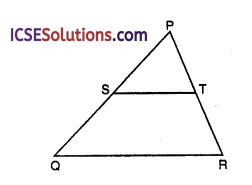(iv) In figure, D and E are the mid-points of AB and AC. If DE = 7.5 cm, then BC = ………….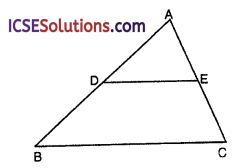(v) Find the value of x in each triangle.Solution:
(i) The line joining the mid-points of two sides of a triangle is parallel to the third side.

(ii) The line drawn through the mid-point of one side of a triangle parallel to another side bisects the third side.

(iii) In figure, S and T are the mid-points of PQ and PR respectively. If ST = 3 cm, then QR = 6 cm.

(iv) In figure, D and E are the mid-points of AB and AC. If DE = 7.5 cm, then BC = 15 cm.

(v) (a) In the given figure,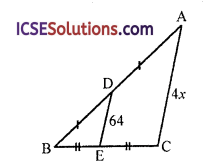In ∆ABC
AD = DB and CE = EB
D and E are the mid points of AB and BC respectively
∴ DE || AC
∴ DE = $$\frac { 1 }{ 2 }$$ AC and DE || AC
64 = $$\frac { 1 }{ 2 }$$ x 4x
⇒ 2x = 64 ⇒ x = $$\frac { 64 }{ 2 }$$ = 32

(b) In ∆ABC,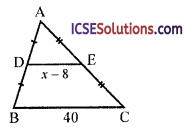AD = DB and AE = EC
∴ DE || BC and
DE = $$\frac { 1 }{ 2 }$$BC
⇒ x – 8 = $$\frac { 1 }{ 2 }$$ x 40 ⇒ x – 8 = 20
⇒ x = 20 + 8 = 28
∴ x = 28

(c) In ∆ABC,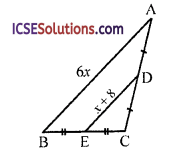AD = DC and BE = EC
∴ DE || AB and DE = $$\frac { 1 }{ 2 }$$ AB
x + 8 = $$\frac { 1 }{ 2 }$$ x 6x ⇒ x + 8 = 3x ⇒ 3x – x = 8
⇒ 2x = 8 ⇒ x = $$\frac { 8 }{ 2 }$$ = 4
∴ x = 4

Question 2.
In ∆ABC, AB = 6 cm, and AC = 3 cm. If M is the mid-point of AB, and a straight line through M parallel to AC cuts BC in N, what is the length of MN?
Solution:
In ∆ABC, AB = 6 cm, AC = 3 cm
M is mid point of AB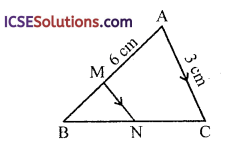A line through M is drawn parallel to AC which cuts BC in N
∵ M is mid point of AB and MN || AC
∴ N is mid point of BC
∴ MN = $$\frac { 1 }{ 2 }$$ AC
= $$\frac { 1 }{ 2 }$$ x 3 cm = 1.5 cm

Question 3.
In the figure, in the ∆ABC, D is the mid-point of AB, E is the mid-point of AC. Calculate:
(i) DE, if BC = 6 cm
(ii) ∠ADE, if ∠DBC = 140°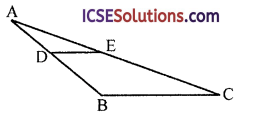Solution:
In the figure,
∆ABC, D is mid-point of AB and E is mid-point of AC
∴ DE || BC and DE = $$\frac { 1 }{ 2 }$$ BC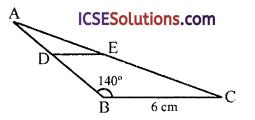(i) ∵ BC = 6 cm
∴ DE = $$\frac { 1 }{ 2 }$$ x 6 = 3 cm

(ii) ∵ ∠DBC = 140°
∵ DE || BC
∴ ∠ADE = ∠DBC or ∠ABC
(corresponding angles)

Question 4.
In figure, D, E, F are the mid-points of BC, CA and AB respectively. Prove that AD bisects EF.Solution:
Given : D, E and F are the mid-points of the sides BC, CA and AB respectively AD and EF are joinedTo prove : AD bisects EF
Construction : Join ED and FD
Proof: In ∆ABC
E and F are the mid-points of CA and AB 1
∴ EF || BC and EF = $$\frac { 1 }{ 2 }$$ BC
Similarly we can prove that
ED || AB and equal to $$\frac { 1 }{ 2 }$$ AB
and FD || AC and equal to $$\frac { 1 }{ 2 }$$ AC
∴ ∆EDF is a parallelogram
∵ The diagonals bisect each other

Question 5.
In figure, ABC is an isosceles triangle in which AB = AC. D, E and F are respectively the mid-points of sides BC, AB and CA. Show that the line segments AD, EF bisect each other at right angles.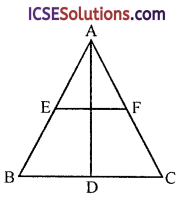Solution:
Given : ∆BC is an isosceles triangle in which AB = AC
D, E and F are the mid-points of BC, AB and AC respectivelyTo prove : AD and EF are perpendicular to each other
Proof: ∵ E and F are mid-points of AB and AC
∴ AE = EB and AF = FC
But AB = AC (given)
∴ AE = AF
and EF || BC and EF = $$\frac { 1 }{ 2 }$$ BC
Now in ∆ABD and ∆ACD
AB = AC (given)
BD = DC (D is mid-point)
∴ ∆ABD = ∆ACD (SSS axiom)
Now in ∆AEG and ∆AFG
AG = AG (common)
AE = AF ($$\frac { 1 }{ 2 }$$ of equal sides)
∴ ∆AEG ≅ ∆AFG (SAS axiom)
∴ ∠AGE = ∠AGF (c.p.c.t.)
But ∠AGE + ∠AFG = 180° (Linear pair)
∴ ∠AGE = ∠AGF = 90°
∴ AD and EF are perpendicular to each other
Hence proved.Question 6.
Prove that the four triangles formed by joining the pairs of mid-points of the three sides of a triangle are congurent to each other.
Solution:
Given : In ∆ABC, D, E and F are the mid-points of the sides BC, CA and AB respectively
DE, EF and FD are joined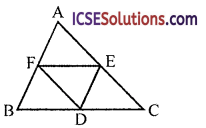To prove : ∆AEF ≅ ∆BDF
∆DEF ≅ ∆CDE
Proof: ∵ D and E are the mid-points of side BC and CA respectively
∴ DE || AB and DE = $$\frac { 1 }{ 2 }$$ AB
∴ DE = BF = FA … (i)
Similarly, D and F are the mid-points of BC andAB respectively
∴ DF || AC and DF = –$$\frac { 1 }{ 2 }$$ AC … (ii)
DF = AE = EC
Now in ∆AEF and ∆DEF
AF = DE (proved)
AE = DF (proved)
EF = EF (common)
∴ ∆AEF ≅ ∆DEF … (i) (SSS criteria)
Similarly we can prove that
∆BDF ≅ ∆DEF … (i)
and ∆CDE ≅ ∆DEF … (ii)
From (i), (ii) and (iii)
∆AEF ≅ ∆BDF ≅ ∆CDE ≅ ∆DEF
Hence proved.

Question 7.
D, E and F are respectively the mid-points of the sides BC, CA and AB of an equilateral triangle ABC. Prove that ∆DEF is also an equilateral triangle.
Solution:
Given : ∆ABC is an equilateral triangle. D, E and F are the mid-points of sides BC, CA and AB respectively
DE, EF and FD are joinedTo prove : ∆DEF is an equilateral triangle
Proof:
∵ E and F are the mid-points of AC and AB respectively
∴ EF || BC and EF = $$\frac { 1 }{ 2 }$$ BC … (i)
Similarly, D and E are the mid-points of BC and AC respectively
∴ DE || AB and DE = $$\frac { 1 }{ 2 }$$ AB … (ii)
and D and F are the mid-points of BC and AB respectively
∴ DF || AC and DF = $$\frac { 1 }{ 2 }$$ AC … (iii)
From (i), (ii) and (iii)
∵ AB = BC = CA (sides of equilateral ∆ABC)
∴$$\frac { 1 }{ 2 }$$ EF = $$\frac { 1 }{ 2 }$$ DE = $$\frac { 1 }{ 2 }$$ DF
⇒ DE = EF = FD
∴ ∆DEF is an equilateral triangle
Hence proved.

Question 8.
In figure, AD and BE are the medians of ∆ABC and BE || DF. Prove that CF = $$\frac { 1 }{ 4 }$$ AC.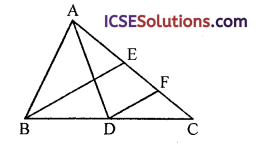Solution:
Given: In AABC, AD and BE are its median BE || DF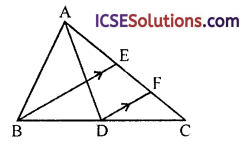To prove : CF = $$\frac { 1 }{ 4 }$$ AC
Proof:
∵ BE is the median
∴ E is mid-point of AC
∴ AE = EC or EC = $$\frac { 1 }{ 2 }$$ AC … (i)
In ∆BCE,
D is mid-point of BC and DF || BE
∴ F is mid-point of EC
∴ FC = $$\frac { 1 }{ 2 }$$ EC … (iv)
From (i) and (ii),
CF = $$\frac { 1 }{ 2 }$$ EC = $$\frac { 1 }{ 2 }$$ ($$\frac { 1 }{ 2 }$$ AC) = $$\frac { 1 }{ 4 }$$AC
Hence CF = $$\frac { 1 }{ 4 }$$ AC
Hence proved.Question 9.
In the given figure, ABC is an isosceles A with AB = AC and CP || BA and AP is the bisector of ext. ∠CAD of ∆ABC. Prove that
(i) ∠PAC = ∠BCA
(ii) ∆BCP is a parallelogram.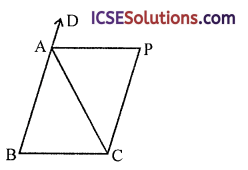Solution:
Given : ∆ABC is an isosceles triangle in which AB = AC
CP || BA is drawn and AP is the bisector of ∠CAD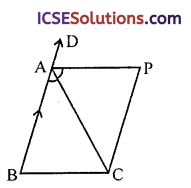To prove :
(i) ∠PAC = ∠BCA
(ii) ABCP is a parallelogram Proof: In ∆ABC,
(i) ∵ AB=AC (given)
∴ ∠C = ∠B (Angles opposite to equal sides)
But Ext. ∠CAD = ∠B + ∠C= 2∠C … (i)
(Sum of its interior opposite angles)
and ∠PAC = ∠PAD = $$\frac { 1 }{ 2 }$$ ∠CAP … (ii)
From (i) and (ii),
∠C = ∠CAP or ∠PAC = ∠BCA

(ii) ∵∠PAC = ∠BCA (proved)
But these are alternate angles
∴ AP || BC
But CP || BA
∴ ABCP is a parallelogram
Hence proved.

Question 10.
ABCD is a kite having AB = AD and BC = CD. Prove that the figure formed by joining the midpoints of the sides, in order, is a rectangle.
Solution:
Given: In the figure,ABCD is a kite in which AB = AD and BC = DC
P, Q, R and S are the mid-points of the sides AB, AD, DC and BC respectively
PQ, QR, RS and PS are joined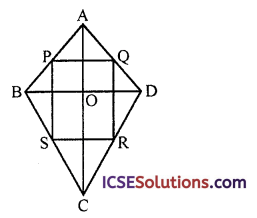To prove : PQRS is a rectangle
Construction : Join BD and AC
Proof: In ∆ABD,
∵ P and Q are mid-points of AB and AD respectively
∴ PQ || BD and PQ = $$\frac { 1 }{ 2 }$$ BD … (i)
Similarly in ABCD,
S and R are the mid-points of BC and DC
∴ SR || BD and SR = $$\frac { 1 }{ 2 }$$ BD … (ii)
From (i) and (ii)
∴ PQ = SR and PQ || SR
Similarly PS = QR and PS || QR
∴ PQRS is a parallelogram
∵ Diagonals AC and BD intersect
Each other at right angles
∴ PQRS is a rectangle
Hence proved.

Question 11.
Show that the quadrilateral, fonned by joining the mid-points of the consecutive sides of a rectangle, is a rhombus.
Solution:
Given : ∆BCD is a rectangle. P, Q, R, and S are the mid-points of the sides AB, BC, CD and DA respectively PQ, QR, RS and SP are joined
To prove : PQRS is a rhombus
Construction : Join PR and SQ, AC and BD are also joined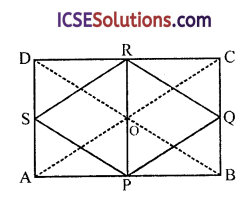Proof: In ∆ABC,
∵ P and Q are mid-points of AB and BC respectively
∴ PQ || AC and PQ = $$\frac { 1 }{ 2 }$$ AC … (i)
∴ S and R are the mid-points of AD and CD respectively
∴ SR || AC and SR = $$\frac { 1 }{ 2 }$$AC … (ii)
From (i) and (ii)
PQRS is a parallelogram
Similarly PS || BD and SP = $$\frac { 1 }{ 2 }$$ BD 1
and QR || BD and QR = $$\frac { 1 }{ 2 }$$ BD
But in rectangle ABCD diagonals AC and BD are equal
∴ PQRS is a rhombus
Hence proved.Question 12.
Quad. ABCD is a rhombus and P, Q, R, S are the mid-points of AB, BC, CD, DA respectively. Prove that quad. PQRS is a rectangle.
Solution:
Given : ABCD is a quad, which is a rhombus. P, Q, R and S are the mid-points of the sides AB, BC, CD and DA respectively PQ, QR, RS and SP are joined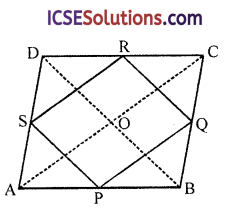To prove : PQRS is a rectangle
Construction : Join AC and BD
Proof: In ∆ABC
∵ P and Q are the mid-points of AB and CD respectively
∴ PQ || AC and PQ = $$\frac { 1 }{ 2 }$$ AC … (i)
R and S are the mid-points of CD and DA respectively
∴ RS || AC and RS = $$\frac { 1 }{ 2 }$$ AC … (ii)
∴ PQRS is a parallelogram
∵ Diagonals of rhombus bisect each other at right angles PQRS is a rectangle
Hence proved.

Question 13.
In the quadrilateral ABCD the mid-points of AB, BC, CD, DA are L, M, P, Q.
(i) Using the mid-point theorem make a statement concerning the lengths and directions of LM and AC.
(ii) Prove that LMPQ is a parallelogram.
(iii) If it is also given that the diagonals AC and BD are equal. What further statement can be made about the parallelogram LMPQ ?
Solution:
Given : In quadrilateral ABCD, L, M, Pand Q are the mid-points of the sides AB, BC, CD and DA respectively. LM and AC are joined.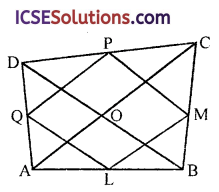To prove :
(i) Using the mid point theorem, make a statement concerning the lengths and directions of LM and AC

(ii) Prove that LMPQ is a parallelogram.

(iii) If it is also given that the diagonals AC and BD are equal what further statement can be made about the parallelogram LMPQ ?
Construction : Join BD, MP, PQ and QL
Proof:
(i) ∵ L and M are the mid-points of AB and BC respectively
∴ LM || AC and LM = $$\frac { 1 }{ 2 }$$ AC … (i)

(ii) Similarly P and Q are the mid-points of CD and DA respectively
∴ PQ || AC and PQ = $$\frac { 1 }{ 2 }$$ AC … (ii)
From (i) and (ii),
LMPQ is a parallelogram

(iii) If diagonals AC and BD are equal
Then ABCD can be a parallelogram or a rectangle
Then LM = MP = PQ = QL Then LMPQ will be a rhombus
(∵ If the sides of a parallelogram are equal then it is a rhombus)

Question 14.
ABCD is a parallelogram E is the mid-point of AB and F is the mid-point of CD. GH is any line that intersects AD, EF and BC in G, P and H respectively. Prove that GP = PH.Solution:
Given : In the figure ABCD is a parallelogram. E and F are the mid-points of AB and CD respectively GH is any line which intersects AD in G, EF in P and BC in H
To prove : GP = PH
Proof:
∵ E and F are the mid-points of AB and DC respectively
∴ EF || AD || BC (∵ ABCD is a parallelogram)
∴ AE = EB ⇒ $$\frac { AE }{ EB }$$ = 1
∴ $$\frac { GP }{ PH }$$ = 1 ⇒ GP = PH (intercept theorem)
Hence proved.

Question 15.
Prove that in a parallelogram, the lines joining a pair of opposite vertices to the mid-points of a pair of opposite sides trisect a diagonal.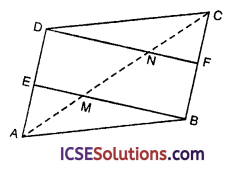Solution:
Given : In ||gm ABCD, E and F are the mid-points of side AD and BC respectively
EB and DF are joined which intersect diagonal AC at M and N
To prove : M and N trisect AC
i.e. AM = MN = NC
Proof: ∵ E and F are the mid-points of AD and BC respectively
∴ BE || DF
∵ EM || DN and AE = ED
AM = MN … (i)
Similarly in ∆BCM,
∵ CF = FB
∴ CN = NM … (ii)
From (i) and (ii),
AM = MN = NC
Hence M and N trisect AC
Hence proved.

Question 16.
In figure, E is the mid-point of side AD of a trapezium ABCD, with AB || DC. A line through E parallel to AB, meets BC in F. Show that F is the mid-point of BC.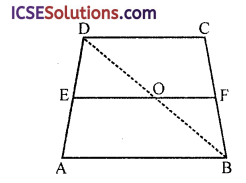Solution:
Given : In trapezium ABCD, AB || DC